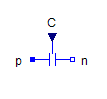Multiphase Ideal Transformer - MapleSim Help

Multiphase Ideal Transformer

Multiphase ideal electrical transformerDescription The Multiphase Ideal Transformers (or Ideal Transformer) component contain $m$ single-phase ideal transformer models, each connected to corresponding phases of ${\mathrm{plug}}_{\mathrm{p1}}$, ${\mathrm{plug}}_{\mathrm{p2}}$, ${\mathrm{plug}}_{\mathrm{n1}}$, and ${\mathrm{plug}}_{\mathrm{n2}}$.Connections

 Name Description Modelica ID ${\mathrm{plug}}_{\mathrm{p1}}$ Port 1 positive $m$-phase plug plug_p1 ${\mathrm{plug}}_{\mathrm{p2}}$ Port 2 positive $m$-phase plug plug_p2 ${\mathrm{plug}}_{\mathrm{n2}}$ Port 1 negative $m$-phase plug plug_n2 ${\mathrm{plug}}_{\mathrm{n1}}$ Port 2 negative $m$-phase plug plug_n1Parameters

 Name Default Units Description Modelica ID $m$ $3$ Number of phases m $n$ $\mathrm{fill}\left(1,m\right)$ $1$ Turns ratio n $\mathrm{Consider Magnetization}$ $\mathrm{false}$ True (checked) means include magnetizing inductance considerMagnetization ${L}_{\mathrm{m1}}$ $\mathrm{fill}\left(1,m\right)$ $H$ Magnetization inductances w.r.t. primary side Lm1Modelica Standard Library The component described in this topic is from the Modelica Standard Library. To view the original documentation, which includes author and copyright information, click here.